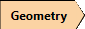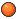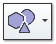RSWebinar: Designing Cost-Effective Ground Improvement Solutions with Settle3 | June 7th, 2023 Register Now

# Sphere

To generate a sphere geometry:

1. Select the Geometryworkflow tab.
2. Select the Sphereoption from the 3D Primitive Geometryoption from the toolbar or sub-menu in the Geometry menu.
3. A dialog should appear. Enter the Center and Radius of your sphere.
4. Select the method for approximating the sphere shape:
• UV Sphere: The method is based on dividing a sphere into vertical and horizontal segments to generate the approximate curvature using planes. This is similar to the longitude and latitude lines on an earth globe. Define the desired number of Subdivisions to define the number of vertical lines in the approximation. Define the desired number of Stacks to define the number of horizontal lines in the approximation.
• Icosphere: The method is based on generating a sphere from a base polyhedron. You can select to use a Tetrahedron, Octahedron, or Icosahedron. If you define a Recursion Level of 1 you will be able to see the base polyhedron. As you increase the Recursion Level, the base shape is subdivided as to increase the number of triangular faces used to approximate the curvature of a sphere.

In the following figure, the sphere is generated using the UVSphere method on the left and Icosphere method on the right.